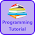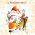# Programming Videos

## Search

### C, C++ Program to Reverse a String without using Strrev Function

Write a C , C++ program to reverse a string without using a string reverse function.  Given a input string, we have to reverse a string without using any in-built functions.

Program to reverse a number

Algorithm to Reverse a String

1.  Initialize a variable.

2. Take a input from user.

3. Count the length of a input string, As we are not using any in-built function.

4. Swap the position of an element.

## C Program to Reverse a String

```#include <stdio.h>

int main() {

char str,  temp;
int i, j = -1;

// Take string input from user.

printf("Enter string");
gets(str);

i = 0;

// count the length of a input string.

while(str[++j]!='\0');

j = j - 1;

/* Swap the positions of an element. */

while (i < j) {
temp = str[i];
str[i] = str[j];
str[j] = temp;
i++;
j--;
}

printf("Reverse of a input string is :%s", str);
return (0);
}

```
Output

Enter string :reverse string

## Reverse a String using While Loop

```#include <stdio.h>

int main()
{

char str[] = "Reverseme";

char reverse;

int i=-1;
int j=0;

//Traverse a String Until it not reach at the end of string

while(str[++i]!='\0');

while(i >= 0)
reverse[j++] = str[--i];

reverse[j] = '\0';

printf("Reverse of  a string is %s",reverse);

return 0;
}

```
Output -

Reverse of a string is emesreveR

## C++ Program to Reverse a String

```#include <iostream.h>

int main()
{

char str[] = "Reverseme";

char reverse;

int i=-1;
int j=0;

/* Count the length, until it each at the end of string. */

while(str[++i]!='\0');

while(i>=0)
reverse[j++]=str[--i];

reverse[j]='\0';

cout<<"Reverse of  a string is"<< reverse;

return 0;

}```

Programming questions on Strings

Programming questions on Recursion

Programming questions on Array

Programming questions on Java

1.thanx....good program!!

2.Nice post

3.Really Awesome Dude, Thanks for your help.

4.5.nice one

6.Thanks for helping us. Can you write a program to reverse a string in java.

1.This comment has been removed by the author.

2.7.#include
#include
#include
void main()
{
clrscr();
char str;
int i=0,k=0;
cout<<"enter any string"<<"\n";
gets(str);
for(i=0;str[i]!='\0';i++);
k=i;
while(k>=0)
{
putchar(str[k--]);
}
getch();
}

8.Thanks for help